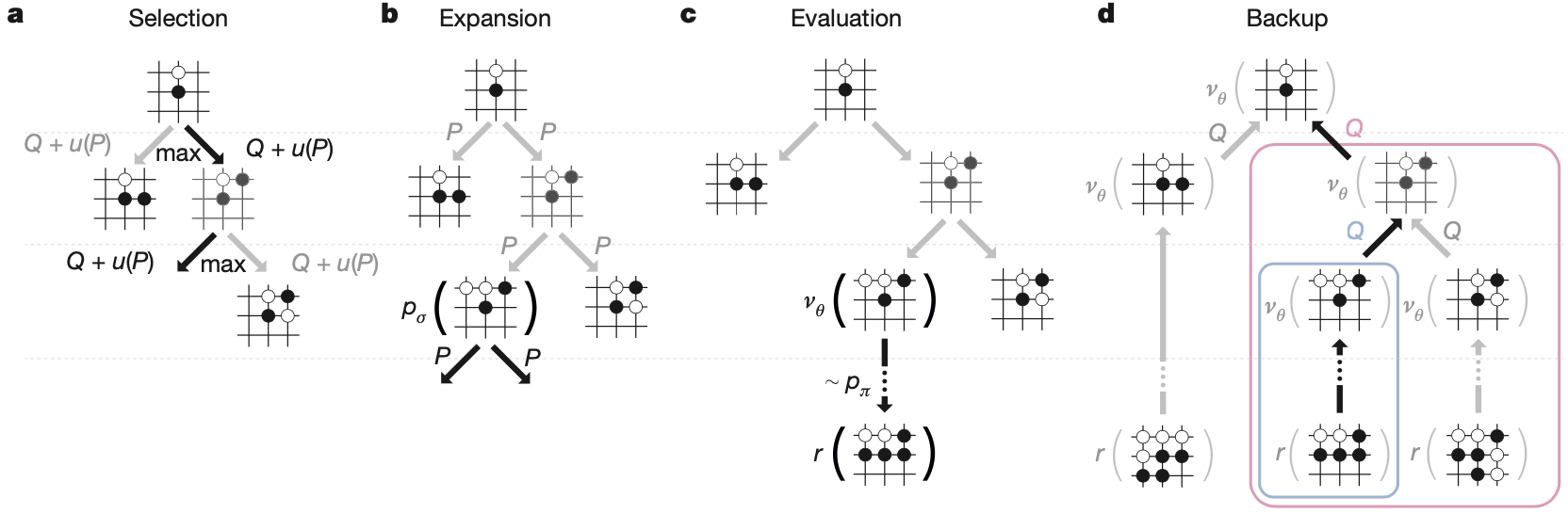# AlphaGo的蒙特卡洛搜索这篇我们来详细讲讲AlphaGo的蒙特卡洛搜索。AlphaGo的智能体现在两个地方：一个是基于现有的深度学习和强化学习技术学习，通过自对弈和专家经验学习到的策略网络和值网络；另一个则是体现在实战阶段，AlphaGo并没有直接应用策略网络包含的知识进行对弈，而是将训练好的策略网络和值网络结合到蒙特卡洛搜索中，实现前瞻性的决策。我们先来捋顺一下AlphaGo中蒙特卡洛搜索的过程，然后解释下这种搜索的合理性。
蒙特卡洛搜索依托的数据结构首先是一棵树，树的根节点是当前AlphaGo需要进行决策的棋盘的状态s。这里需要注意的是，AlphaGo在决策每个落子的时候都会重新运行搜索，相应的也会重新建一棵搜索树。
我们考虑像这样的一棵搜索树都需要哪些量来确定呢？首先，根节点的孩子节点是什么呢？应该是棋盘的每一个可能的次状态，也就是说此时此刻每一个合法的落子都应该对应一个孩子节点。但是次状态太多，全部扩展的话，树的深度会受到限制。在蒙特卡洛搜索中，会根据指标选择一个动作作为对当前状态的扩展，扩展到新的状态后，再根据这个定量的指标选择一个新的动作，使用的定量指标如下

a_t = \arg\max_a(Q(s_t,a)+u(s_t,a)).

接下来我们来说这个搜索树的边存储的变量以及相应的评估方式。搜索树的每条边都存储三个变量：Q(s_t,a)u(s_t,a)N(s_t,a)。其中，N(s_t,a) 表示在所有的 n 次模拟中，(s_t,a) 这条边被访问的次数，而 Q(s_t,a)u(s_t,a)都是在这个次数的基础上定义的统计量。 Q(s_t,a) 表示沿着当前路径扩展搜索树获胜的数学期望，以获得的奖励的均值来统计

Q(s,a)=\frac{1}{N(s,a)}\sum_{i=1}^{n}1(s,a,i)V(s_L^i).

1(s,a,i) 表示在第 i 次模拟中 (s,a) 这条边是否被使用过，而每条边的 N(s,a) 正是 \sum_{i=1}^{n}1(s,a,i)。所以，Q(s,a) 表示的正是奖励的期望。公式中的 V(s_L^i) 指的是第 i 次模拟得到的奖励值，s_L^i 则表示该次模拟到树的第 L 层停止后对应的叶节点，V(s_L^i) 由两部分组成

V(s_L)=(1-\lambda)v_\theta(s_L)+\lambda z_L.

v_\theta(s_L) 是基于已经训练好的值网络的一次快速评估，评估在状态 s_L 下获胜的数学期望，z_L 是使用训练好的轻量级策略网络 p_\pi 进行快速的连续的决策，从而在短时间内从状态 s_L 到达某一方获胜的终止状态 s_T 后得到的奖励值。
在刚开始的时候，每个可能的次状态的 Q(s,a) 都是 \infty，因为 N(s,a) = 0。所以一开始就是随机选择一个，第一次模拟结束后，Q(s,a) 就不是 \infty 了，继而其他的次状态会被继续选中进行模拟，当众多状态都被模拟过一遍时候，就开始进入公平竞争了，谁的 Q 值大，谁被遍历的次数就多。
u(s,a) 是bonus

u(s,a) \propto \frac{P(s,a)}{1+N(s,a)},

在完成所有的 n 次遍历后，我们选择被访问次数最多的动作执行即可。